### Displacement and Strain: The Displacement Gradient Tensor

Another three dimensional measure of deformation is the displacement gradient tensor. The displacement gradient tensor appears naturally when we attempt to write the relationship between a tangent vector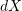in the reference configuration deformation and its image under deformation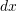such that: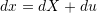where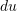is the “displacement” vector that describes the change in tangent vectors.

As discussed in the deformation gradient section,andare related as follows: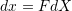Therefore, the “displacement” vectorcan be written as: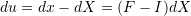The tensor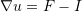is denoted the displacement gradient tensor and can be written in component form as follows: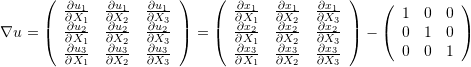As described in the skewsymmetric tensors section, every tensor can be uniquely decomposed into two additive components, a symmetric tensor and a skewsymmetric tensor. By denoting the symmetric part asor the “infinitesimal strain tensor” and the skewsymmetric part as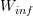or the “infintesimal rotation tensor” we can write the relationship between the vectors in the reference and deformed configuration as follows: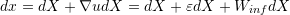In other words, the additive decomposition of the displacement gradient tensor allows to write the deformed vectoras the additive combination of three vectors: the original vector, plus a “strain” or “stretch” component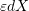, plus a “rotation” component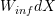. The stretch component can be calculated using the symmetric tensorwhile the rotation component can be calculated using the skewsymmetric tensor. Both tensors are physically meaningful when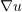has very small components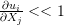(small displacements).